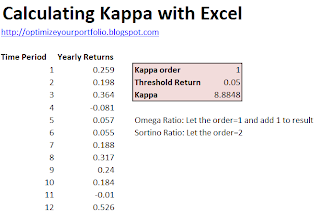# Calculate Kappa with Excel

Introduced by Kaplan and Knowles (2004), Kappa unifies both the Sortino Ratio and the Omega Ratio, and is defined by the following equation.where μ is the mean return, τ is the threshold return, and LPMn is the n-th order Lower Partial Moment.

The Sortino Ratio and Omega Ratio are simply variants of Kappa.  The Sortino Ratio is K2(τ) and the Omega Ratio is K1(τ) + 1.Kaplan and Knowles (2004) conclude that while higher Kappa values are better, the asbolute differences of Kappa between different investments are not easily interpreted; accordingly, Kappa should only be used to rank investments.  This paper describes the theory and applications of Kappa in greater detail.

The Excel spreadsheet calculates Kappa from discrete return data (although you could also calculate Kappa from the moments of a probability distribution).  The formula implemented in the Excel spreadsheet is relatively simple. If returns is a named range containing the returns, tau is the threshold return desired by the investor and n is the Kappa order, then Kappa is given by

{=AVERAGE(returns-tau)/AVERAGE( IF(returns < tau,1,0)*(tau-returns)^n)^(1/n)}

where the curly braces {} signify a matrix formula entered by Ctrl+Shift+Enter.